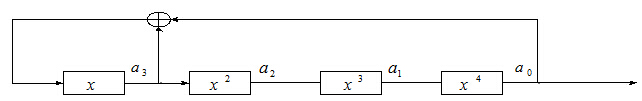# 线性反馈移位寄存器与梅森旋转算法

（1）级，每一级包含一个比特，比如11010110是一个8级的移位寄存器产生的

（2）反馈函数，线性反馈移位寄存器的反馈函数是线性的，非线性反馈移位寄存器的反馈函数是非线性的MT19937-32只需要用到32位，那么为什么要选择周长为的算法呢？ 那是因为这样做随机性很好。

#include <iostream>
#include <string.h>
#include <stdio.h>
#include <time.h>

using namespace std;

bool isInit;
int index;
int MT;  //624 * 32 - 31 = 19937

void srand(int seed)
{
index = 0;
isInit = 1;
MT = seed;
//对数组的其它元素进行初始化
for(int i = 1; i < 624; i++)
{
int t = 1812433253 * (MT[i - 1] ^ (MT[i - 1] >> 30)) + i;
MT[i] = t & 0xffffffff;   //取最后的32位赋给MT[i]
}
}

void generate()
{
for(int i = 0; i < 624; i++)
{
// 2^31 = 0x80000000
// 2^31-1 = 0x7fffffff
int y = (MT[i] & 0x80000000) + (MT[(i + 1) % 624] & 0x7fffffff);
MT[i] = MT[(i + 397) % 624] ^ (y >> 1);
if (y & 1)
MT[i] ^= 2567483615;
}
}

int rand()
{
if(!isInit)
srand((int)time(NULL));
if(index == 0)
generate();
int y = MT[index];
y = y ^ (y >> 11);                 //y右移11个bit
y = y ^ ((y << 7) & 2636928640);   //y左移7个bit与2636928640相与，再与y进行异或
y = y ^ ((y << 15) & 4022730752);  //y左移15个bit与4022730752相与，再与y进行异或
y = y ^ (y >> 18);                 //y右移18个bit再与y进行异或
index = (index + 1) % 624;
return y;
}

int main()
{
srand(0);  //设置随机种子
int cnt = 0;
for(int i = 0; i < 1000000; i++)
{
if(rand() & 1)
cnt++;
}
cout<<cnt / 10000.0<<"%"<<endl;
return 0;
}©️2019 CSDN 皮肤主题: 技术黑板 设计师: CSDN官方博客